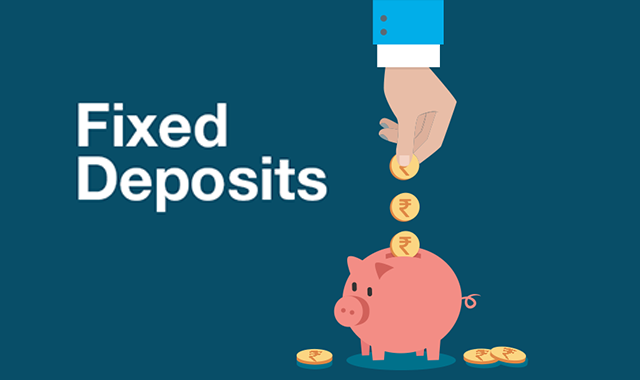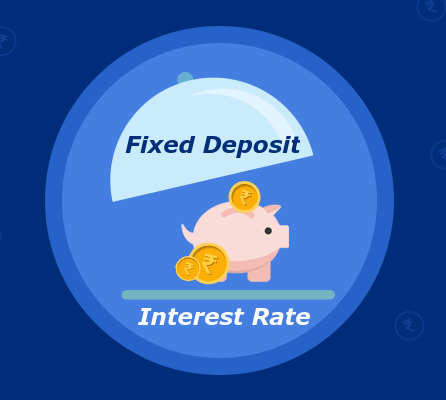Fixed deposit is a highly popular investment option which lets investors earn appreciable amount on a fixed amount more than a savings account. Depositors can save a lump sum amount at one go. The profit made on the fixed amount is determined by the interest rate provided by the institution at which the deposit is being booked.

Before investing in a fixed deposit it is however wise to evaluate the return on your investment. This can be done using the FD calculator which is available an online.

Let’s know More About the FD Calculator –

There are three major components of the calculator –

• Principal amount — this is the lump sum amount for which the deposit is booked for. The interest that you make on your deposit is directly proportional to its principal amount. This simple means, higher the principal amount, greater is the interest earned.
• Rate of interest — this is predetermined by the bank. The interest income and the maturity amount are directly proportional to the FD interest rate, i.e., higher is the interest rate, higher will be the interest income and its maturity amount.
• Tenure — The interest accumulated and the maturity amount on the deposit depend on its tenure. So, higher the tenure, the higher will be the interest and its subsequent maturity amount.

Know about Types of Fixed Deposit

Usually, the interest rate provided by banks are calculated in two ways –

• Simple Interest — Where, the principal amount is multiplied with the annual rate of interest and the tenure (in years)
• Compound Interest — Where, Interest amount earned is calculated on the principal amount as well as the interest, in a defined time period.It may be noted that to make the most from an FD as an investor you must opt for higher FD interest rates. The deposit can be renewed at regular intervals too to avail top up rate.

Formula for Calculating FD Interest Rate

The formula used for calculating FD interest rate is:

A = P (1 + r/25) 4n

Where, A is the maturity amount, P is the deposit amount and n is the frequency of compounded interest. Using this formula, you can know the final maturity amount.While, senior citizens can book fixed deposit with 0.5% higher rate than regular deposits. This allows them to accumulate wealth and meet their financial goals even at a time in their lives when they are not working.

Besides, the entities who can book an FD are –

• Hindu Undivided Family (HUF)
• Sole Proprietorships, Partnership Firms, and Companies Including Group Companies
• Clubs, Associations, and Societies
• Family Trusts

Nowadays, fixed deposit can be easily applied either in your name or for your family members and even children. The process requires minimum paperwork and can be booked online to help you meet your financial goals.